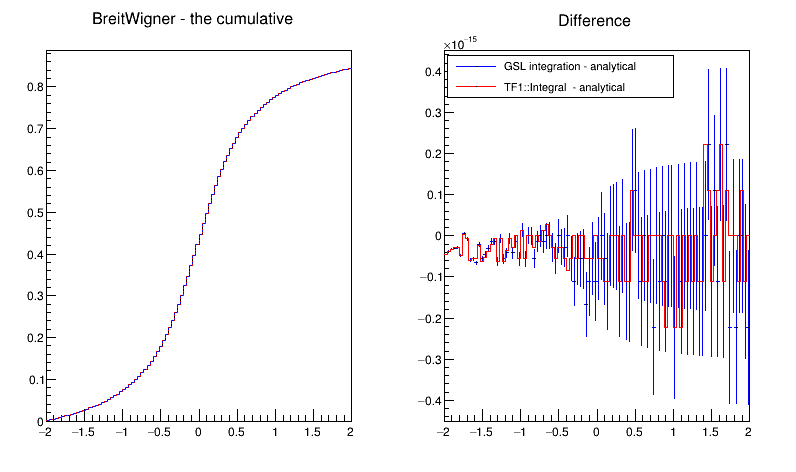# mathmoreIntegration¶

Example on the usage of the adaptive 1D integration algorithm of MathMore it calculates the numerically cumulative integral of a distribution (like in this case the BreitWigner) to execute the macro type it (you need to compile with AClic)

root .x mathmoreIntegration.C+


This tutorials require having libMathMore built with ROOT.

To build mathmore you need to have a version of GSL >= 1.8 installed in your system The ROOT configure will automatically find GSL if the script gsl-config (from GSL) is in your PATH,. otherwise you need to configure root with the options --gsl-incdir and --gsl-libdir.

Author: M. Slawinska, L. Moneta
This notebook tutorial was automatically generated with ROOTBOOK-izer from the macro found in the ROOT repository on Wednesday, August 17, 2022 at 09:34 AM.

In :
%%cpp -d
#include "TMath.h"
#include "TH1.h"
#include "TCanvas.h"
#include "TLegend.h"

/*#include "TLabel.h"*/
#include "Math/Functor.h"
#include "Math/WrappedFunction.h"
#include "Math/IFunction.h"
#include "Math/Integrator.h"
#include <iostream>

#include "TStopwatch.h"
#include "TF1.h"

#include <limits>

int nc = 0;


!calculates exact integral of Breit Wigner distribution !and compares with existing methods

In :
%%cpp -d
double exactIntegral( double a, double b) {

return (TMath::ATan(2*b)- TMath::ATan(2*a))/ TMath::Pi();
}


Definition of a helper function:

In :
%%cpp -d
double func( double x){
nc++;
return TMath::BreitWigner(x);
}


TF1 requires the function to have the ( )( double , double ) signature

In :
%%cpp -d
double func2(const double *x, const double * = 0){
nc++;
return TMath::BreitWigner(x);
}


Definition of a helper function:

In :
%%cpp -d
void  testIntegPerf(double x1, double x2, int n = 100000){

std::cout << "\n\n***************************************************************\n";
std::cout << "Test integration performances in interval [ " << x1 << " , " << x2 << " ]\n\n";

TStopwatch timer;

double dx = (x2-x1)/double(n);

//ROOT::Math::Functor1D<ROOT::Math::IGenFunction> f1(& TMath::BreitWigner);
ROOT::Math::WrappedFunction<> f1(func);

timer.Start();
ROOT::Math::Integrator ig(f1 );
double s1 = 0.0;
nc = 0;
for (int i = 0; i < n; ++i) {
double x = x1 + dx*i;
s1+= ig.Integral(x1,x);
}
timer.Stop();
std::cout << "Time using ROOT::Math::Integrator        :\t" << timer.RealTime() << std::endl;
std::cout << "Number of function calls = " << nc/n << std::endl;
int pr = std::cout.precision(18);  std::cout << s1 << std::endl;  std::cout.precision(pr);

//TF1 *fBW = new TF1("fBW","TMath::BreitWigner(x)",x1, x2);  //  this is faster but cannot measure number of function calls
TF1 *fBW = new TF1("fBW",func2,x1, x2,0);

timer.Start();
nc = 0;
double s2 = 0;
for (int i = 0; i < n; ++i) {
double x = x1 + dx*i;
s2+= fBW->Integral(x1,x );
}
timer.Stop();
std::cout << "Time using TF1::Integral :\t\t\t" << timer.RealTime() << std::endl;
std::cout << "Number of function calls = " << nc/n << std::endl;
pr = std::cout.precision(18);  std::cout << s1 << std::endl;  std::cout.precision(pr);

}


Definition of a helper function:

In :
%%cpp -d
void  DrawCumulative(double x1, double x2, int n = 100){

std::cout << "\n\n***************************************************************\n";
std::cout << "Drawing cumulatives of BreitWigner in interval [ " << x1 << " , " << x2 << " ]\n\n";

double dx = (x2-x1)/double(n);

TH1D *cum0 = new TH1D("cum0", "", n, x1, x2); //exact cumulative
for (int i = 1; i <= n; ++i) {
double x = x1 + dx*i;
cum0->SetBinContent(i, exactIntegral(x1, x));

}

// alternative method using ROOT::Math::Functor class
ROOT::Math::Functor1D f1(& func);

TH1D *cum1 = new TH1D("cum1", "", n, x1, x2);
for (int i = 1; i <= n; ++i) {
double x = x1 + dx*i;
cum1->SetBinContent(i, ig.Integral(x1,x));
}

TF1 *fBW = new TF1("fBW","TMath::BreitWigner(x, 0, 1)",x1, x2);

TH1D *cum2 = new TH1D("cum2", "", n, x1, x2);
for (int i = 1; i <= n; ++i) {
double x = x1 + dx*i;
cum2->SetBinContent(i, fBW->Integral(x1,x));
}

TH1D *cum10 = new TH1D("cum10", "", n, x1, x2); //difference between  1 and exact
TH1D *cum20 = new TH1D("cum23", "", n, x1, x2); //difference between 2 and excact
for (int i = 1; i <= n; ++i) {
double delta  =  cum1->GetBinContent(i) - cum0->GetBinContent(i);
double delta2 =  cum2->GetBinContent(i) - cum0->GetBinContent(i);
//std::cout << " diff for " << x << " is " << delta << "  " << cum1->GetBinContent(i) << std::endl;
cum10->SetBinContent(i, delta );
cum10->SetBinError(i, std::numeric_limits<double>::epsilon() * cum1->GetBinContent(i) );
cum20->SetBinContent(i, delta2 );
}

TCanvas *c1 = new TCanvas("c1","Integration example",20,10,800,500);
c1->Divide(2,1);
c1->Draw();

cum0->SetLineColor(kBlack);
cum0->SetTitle("BreitWigner - the cumulative");
cum0->SetStats(0);
cum1->SetLineStyle(2);
cum2->SetLineStyle(3);
cum1->SetLineColor(kBlue);
cum2->SetLineColor(kRed);
c1->cd(1);
cum0->DrawCopy("h");
cum1->DrawCopy("same");
//cum2->DrawCopy("same");
cum2->DrawCopy("same");

c1->cd(2);
cum10->SetTitle("Difference");
cum10->SetStats(0);
cum10->SetLineColor(kBlue);
cum10->Draw("e0");
cum20->SetLineColor(kRed);
cum20->Draw("hsame");

TLegend * l = new TLegend(0.11, 0.8, 0.7 ,0.89);
l->AddEntry(cum10, "GSL integration - analytical ");
l->Draw();

c1->Update();
std::cout << "\n***************************************************************\n";

}


Arguments are defined.

In :
double a = -2;
double b = 2;

In :
DrawCumulative(a, b);
testIntegPerf(a, b);***************************************************************
Drawing cumulatives of BreitWigner in interval [ -2 , 2 ]

***************************************************************

***************************************************************
Test integration performances in interval [ -2 , 2 ]

Time using ROOT::Math::Integrator        :	0.0883331
Number of function calls = 69
42201.6649413923442
Time using TF1::Integral :			0.458382
Number of function calls = 91
42201.6649413923442